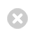Close

MPHY0021: Research Software Engineering With Python

Home# Construction¶

## Construction¶

Software design gets a lot of press (Object orientation, UML, design patterns).

In this session we're going to look at advice on software construction.

### Construction vs Design¶

For a given piece of code, there exist several different ways one could write it:

• Choice of variable names
• Choice of layout

The consideration of these questions is the area of Software Construction.

### Low-level design decisions¶

We will also look at some of the lower-level software design decisions in the context of this section:

• Division of code into subroutines
• Subroutine access signatures
• Choice of data structures for readability

### Algorithms and structures¶

We will not, in discussing construction, be looking at decisions as to how design questions impact performance:

• Choice of algorithms
• Choice of data structures for performance
• Choice of memory layout

We will consider these in a future discussion of performance programming.

### Architectural design¶

We will not, in this session, be looking at the large-scale questions of how program components interact, the stategic choices that govern how software behaves at the large scale:

• Where do objects get made?
• Which objects own or access other objects?
• How can I hide complexity in one part of the code from other parts of the code?

We will consider these in a future session.

### Construction¶

So, we've excluded most of the exciting topics. What's left is the bricks and mortar of software: how letters and symbols are used to build code which is readable.

### Literate programming¶

In literature, books are enjoyable for different reasons:

• The beauty of stories
• The beauty of plots
• The beauty of characters
• The beauty of paragraphs
• The beauty of sentences
• The beauty of words

Software has beauty at these levels too: stories and characters correspond to architecture and object design, plots corresponds to algorithms, but the rhythm of sentences and the choice of words corresponds to software construction.

### Programming for humans¶

• Remember you're programming for humans as well as computers
• A program is the best, most rigorous way to describe an algorithm
• Code should be pleasant to read, a form of scholarly communication

Read Steve McConnell's Code Complete [UCL library].

### Setup¶

This notebook is based on a number of fragments of code, with an implicit context. We've made a library to set up the context so the examples work.

In :
%%writefile context.py
from unittest.mock import Mock, MagicMock
class CompMock(Mock):
def __sub__(self, b):
return CompMock()
def __lt__(self,b):
return True
def __abs__(self):
return CompMock()
array=[]
agt=[]
ws=[]
agents=[]
counter=0
x=MagicMock()
y=None
agent=MagicMock()
value=0
bird_types=["Starling", "Hawk"]
import numpy as np
average=np.mean
hawk=CompMock()
starling=CompMock()
sEntry="2.0"
entry ="2.0"
iOffset=1
offset =1
anothervariable=1
flag1=True
variable=1
flag2=False
def do_something(): pass
chromosome=None
start_codon=None
subsequence=MagicMock()
transcribe=MagicMock()
ribe=MagicMock()
find=MagicMock()
can_see=MagicMock()
my_name=""
your_name=""
flag1=False
flag2=False
start=0.0
end=1.0
step=0.1
birds=[MagicMock()]*2
resolution=100
pi=3.141
result= *resolution
import numpy as np
import math
data= [math.sin(y) for y in np.arange(0,pi,pi/resolution)]
import yaml
import os

Writing context.py